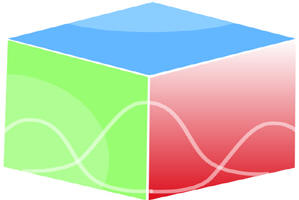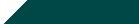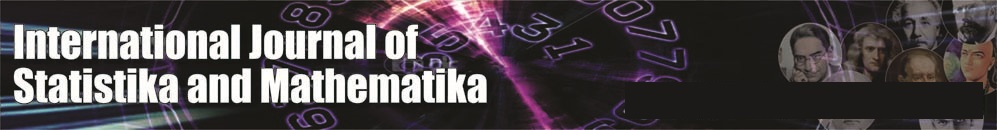``Home| Journals | Statistics Online Expert | About Us | Contact UsUntitled Document

[

Cost- benefit analysis of two dissimilar warm standby system subject to failure due to atmospheric pressure and gravitational force with switch failure

Ashok Kumar Saini

Associate Professor, Department of Mathematics, B. L. J. S. College, Tosham, Bhiwani, Haryana, INDIA.

Email: [email protected]

Research Article

Abstract               Introduction: Development is a multi dimensional process. There are many interrelated and interacting process involved in it – social, economic, political, cultural, educational, technology, etc. Faults in any of the processes affect other processes and hence the overall development. Two-unit standby system subject to environmental conditions such as shocks, change of weather conditions etc. have been discussed in reliability literature by several authors due to significant importance in defence, industry etc. In the present paper we have taken two-non-identical warm standby system with failure time distribution as exponential and repair time distribution as general. The Role of atmospheric pressure and gravitational force under which the system operates plays significant role on its working. We are considering system under (i) atmospheric pressure and (ii) Gravitational force causing different types of failure requiring different types of repair facilities. Using semi Markov regenerative point technique we have calculated different reliability characteristics such as MTSF, reliability of the system, availability analysis in steady state, busy period analysis of the system under repair, expected number of visits by the repairman in the long run and gain-function and graphs are drawn.

Keyword: warm standby, atmospheric pressure, gravitational force, switches failure.

INTRODUCTION

The original concept for the reliability was that a chain cannot be stronger then its weakest link. Work based on this idea gave rise to a great improvement in the theory of reliability. The mathematical theory of reliability has grown out of the demands of modern technology and particularly out of experiences in World War II (1939-1945) with complex military systems although the concept of reliability is as old as man himself.

Assumptions

• The failure time distribution is exponential whereas the repair time distribution is arbitrary of two non-identical units.
• The repair facility is of four types:

Type I, II repair facility

• when failure due to atmospheric pressure and gravitational force of first unit occurs respectively and

Type III, IV repair facility

• when failure due to atmospheric pressure and gravitational force of the second unit occurs respectively.
• The repair starts immediately upon failure of units and the repair discipline is FCFS.
• The repairs are perfect and start immediately as soon as the atmospheric pressure and gravitational force of the system becomes normal. The atmospheric pressure and gravitational force in both the units do not occur simultaneously.
• The failure of a unit is detected immediately and perfectly.
• The switches are perfect and instantaneous.
• All random variables are mutually independent.

Symbols for states of the System

Superscripts: O, WS, SO, FAP, FGF, SFO

Operative, Warm Standby, Stops the operation, failure due to atmospheric pressure, failure due to gravitational force, Switch failed but operable respectively

Subscripts: nap, ap, gf, ur, wr, uR

No atmospheric pressure, atmospheric pressure, gravitational force, under repair, waiting for repair, under repair continued respectively

Up states: 0, 1, 2, 9;

Down states: 3,4,5,6,7,8,10,11

Regeneration point: 0,1,2,4,7,10

States of the System

0(Onap, WSnap)

One unit is operative and the other unit is warm standby and there are no atmospheric pressure and no gravitational force in both the units.

1(SOnap, Onap)

The operation of the first unit stops automatically due to atmospheric pressure and warm standby unit’s starts operating with no atmospheric pressure.

2(FAPur, Onap)

The first unit fails and undergoes repair after the atmospheric pressure are over and the other unit continues to be operative with no atmospheric pressure.

3(FAPuR, SOuap)

The repair of the first unit is continued from state 2 and the operation of second unit stops automatically due to atmospheric pressure.

4(FAPur, SOgf) The first unit fails and undergoes repair after the atmospheric pressure are over and the other unit also stops automatically due to gravitational force.

5(FAPuR, FGFgf,wr) The repair of the first unit is continued from state 4 and the other unit is failed due to gravitational force in it and is waiting for repair.

6(Onap, FGFur) The first unit becomes operative with no atmospheric pressure and the second unit is failed due to gravitational force is under repair.

7(SOngf, SFOnap, ur)

The operation of the first unit stops automatically due to gravitational force and during switchover to the second unit switch fails and undergoes repair and there is no atmospheric pressure.

8(FGFgf, wr, SFOngf, uR)

The repair of failed switch is continued from state 7 and the first unit is failed after gravitational force is waiting for repair.

9(Onap, SOgf)

The first unit is operative with no atmospheric pressure and the operation of warm standby second unit is stopped automatically due to gravitational force.

10(SOgf, SFur)

The operation of the first unit stops automatically due to gravitational force and the second unit switch fails and undergoes repair after the gravitational force is over.

11(FGFgf, wr, FGFuR)

The repair of the second unit is continued from state 10 and the first unit is failed due to gravitational force is waiting for repair.

Figure 1: The State Transition Diagram

TRANSITION PROBABILITIES

Simple probabilistic considerations yield the following expressions:

p01 = , P07 =

p09 = , p12 = , p14 =

P20= G1*( λ1), P22(3) = G1*( λ1)=p23, P72 = G2*( λ4),

P72(8) = G2*( λ4)= P78

We can easily verify that

p01 + p07 + p09 = 1, p12 + p14 = 1, p20 + p23 (=p22(3))= 1, p46(6)= 1 p60 = 1,

p72+ P72(5) + p74 = 1, p9,10 =1, p10,2 + p10,2(11) = 1                                                                                                                       (1)

And mean sojourn time are

µ0 = E(T) =                                                                                                                                                              (2)

Mean Time To System Failure

We can regard the failed state as absorbing

,

(3-5)

Taking Laplace-Stiltjes transform of eq. (3-5) and solving for

= N1(s) / D1(s)                                                                                                                                                                   (6)

Where

N1(s) = {  +

D1(s) = 1 -

Making use of relations (1) and (2) it can be shown that θ0*(0) =1, which implies that θ0(t) is a proper distribution.

MTSF = E[T] = d/ds θ0*(s)       = D1(0) - N1(0)) / D1 (0)

s=0

= ( +p01  + p01 p12  + p09 ) / (1 - p01 p12 p20 )

where

+  + ,  + , + (3),

AVAILABILITY ANALYSIS

Let Mi(t) be the probability of the system having started from state I is up at time t without making any other regenerative state belonging to E. By probabilistic arguments, we have

The value of M0(t), M1(t), M2(t), M4(t) can be found easily.

The point wise availability Ai(t) have the following recursive relations

A0(t) = M0(t) + q01(t)[c]A1(t) + q07(t)[c]A7(t) + q09(t)[c]A9(t)

A1(t) = M1(t) + q12(t)[c]A2(t) + q14(t)[c]A4(t), A2(t) = M2(t) + q20(t)[c]A0(t) + q22(3)(t)[c]A2(t)

A4(t) = q46(3)(t)[c]A6(t), A6(t) = q60(t)[c]A0(t)

A7(t) = (q72(t)+ q72(8)(t)) [c]A2(t) + q74 (t)[c]A4(t)

A9(t) = M9(t) + q9,10(t)[c]A10(t), A10(t) = q10,2(t)[c]A2(t) + q10,2(11)(t)[c]A2(t)                                                                              (7-14)

Taking Laplace Transform of eq. (7-14) and solving for

= N2(s) / D2(s)                                                                                                                                                  (15) Where

N2(s) = (1 -  22(3)(s)) {  0(s) + 01(s) 1(s) + 09(s) 9(s)}+ 2(s){ 01(s) 42(s) + 07(s)72(s) +  73(8)(s)) +  09 (s) 9,10 (s)( 10,2 (s) + 10,2(11)(s))}

D2(s) = (1 -  22(3)(s)) { 1 -  46(5)(s) 60(s) ( 01(s) 44 (s) + 07(s) 74(s))

- 20(s)01(s)12(s)07(s)(  72(s)) +  72(8)(s) +  09 (s) 9,10 (s)

( 10,2 (s) + 10,2(11)(s))}

A0 =  =  =

Using L’ Hospitals rule, we get

A0 =  =                                                                                                                                            (16)

Where

N2(0)= p20(0(0) + p011(0) + p09 9(0) ) + 2(0) (p01p12 + p07 (p72

+ p72(8) + p09 ))

D2(0) = p20{  + p01 + (p01 p14 + p07 p74 )+ p07  + p07  + p09()

+ { 1- ((p01p14 + p07 p74 )}

, ,

The expected up time of the system in (0,t] is

(t) =  So that                                                                                                       (17)

The expected down time of the system in (0,t] is

(t) = t- (t) So that (18)

The expected busy period of the server for repairing the failed unit under gravitational force in (0,t]

R0(t) = S0(t) + q01(t)[c]R1(t) + q07(t)[c]R7(t) + q09(t)[c]R9(t)

R1(t) = S1(t) + q12(t)[c]R2(t) + q14(t)[c]R4(t),

R2(t) = q20(t)[c]R0(t) + q22(3)(t)[c]R2(t)

R4(t) = q46(3)(t)[c]R6(t), R6(t) = q60(t)[c]R0(t)

R7(t) = (q72(t)+ q72(8)(t)) [c]R2(t) + q74 (t)[c]R4(t)

R9(t) = S9(t) + q9,10(t)[c]R10(t), R10(t) = q10,2(t) + q10,2(11)(t)[c]R2(t)                                                                                                (19-26)

Taking Laplace Transform of eq. (19-26) and solving for

= N3(s) / D2(s)                                                                                                                                                                 (27)

Where

N2(s) = (1 -  22(3)(s)) {  0(s) + 01(s) 1(s) + 09(s) 9(s)} and D2(s) is already defined.

In the long run, R0 =                                                                                                                                                        (28)

where N3(0)= p20(0(0) + p011(0) + p09 9(0) ) and D2(0) is already defined.

The expected period of the system under gravitational force in (0,t] is

(t) =  So that

The expected Busy period of the server for repairing the failed units under atmospheric pressure by the repairman in (0,t]

B0(t) = q01(t)[c]B1(t) + q07(t)[c]B7(t) + q09(t)[c]B9(t)

B1(t) = q12(t)[c]B2(t) + q14(t)[c]B4(t), B2(t) = q20(t)[c] B0(t) + q22(3)(t)[c]B2(t)

B4(t) = T4 (t)+ q46(3)(t)[c]B6(t), B6(t) = T6 (t)+ q60(t)[c]B0(t)

B7(t) = (q72(t)+ q72(8)(t)) [c]B2(t) + q74 (t)[c]B4(t)

B9(t) = q9,10(t)[c]B10(t), B10(t) = T10 (t)+ (q10,2(t) + q10,2(11)(t)[c]B2(t)                                                                                              (29- 36)

Taking Laplace Transform of eq. (29-36) and solving for

= N4(s) / D2(s)                                                                                                                                                                  (37)

Where

N4(s) = (1 -  22(3)(s)) { 01(s)14(s) 4(s) + 46 (5)(s) 6(s)) + 07(3)(s) 74(s)( 4(s)

+  46(5)(s) 6(s))+ 09(s)09,10(s)  10(s) )

In steady state, B0 =                                                                                                                                                       (38)

where N4(0)= p20 {( p01 p14 + p07 p74) (4(0) +6(0)) + p09 10(0) } and D2(0) is already defined.

The expected busy period of the server for repair in (0,t] is

(t) =  So that                                                                                                                      (39)

The expected Busy period of the server for repair of switch in (o,t]

P0(t) = q01(t)[c]P1(t) + q07(t)[c]P7(t) + q09(t)[c]P9(t)

P1(t) = q12(t)[c]P2(t) + q14(t)[c]P4(t), P2(t) = q20(t)[c]P0(t) + q22(3)(t)[c]P2(t)

P4(t) = q46(3)(t)[c]P6(t), P6(t) = q60(t)[c]P0(t)

P7(t) = L7(t)+ (q72(t)+ q72(8)(t)) [c]P2(t) + q74 (t)[c]P4(t)

P9(t) = q9,10(t)[c]P10(t), P10(t) = (q10,2(t) + q10,2(11)(t))[c]P2(t)                                                                                                           (40-47)

Taking Laplace Transform of eq. (40-47) and solving for

= N5(s) / D2(s)                                                                                                                                                                   (48)

where N2(s) = 07(s )  7(s) 1 -  22(3)(s)) and D2(s) is defined earlier.

In the long run, P0 =                                                                                                                                                        (49 )

where N5(0)= p20 p07 4(0) and D2(0) is already defined.

The expected busy period of the server for repair of the switch in (0,t] is

(t) =  So that                                                                                                                        (50)

The expected number of visits by the repairman for repairing the non-identical units in (0,t]

H0(t) = Q01(t)[c]H1(t) + Q07(t)[c]H7(t) + Q09(t)[c]H9(t)

H1(t) = Q12(t)[c][1+H2(t)] + Q14(t)[c][1+H4(t)], H2(t) = Q20(t)[c]H0(t) + Q22(3)(t)[c]H2(t)

H4(t) = Q46(3)(t)[c]H6(t), H6(t) = Q60(t)[c]H0(t)

H7(t) = (Q72(t)+ Q72(8)(t)) [c]H2(t) + Q74 (t)[c]H4(t)

H9(t) = Q9,10(t)[c][1+H10(t)], H10(t) = (Q10,2(t)[c] + Q10,2(11)(t))[c]H2(t)                                                                          (51-58)

Taking Laplace Transform of eq. (51-58) and solving for

= N6(s) / D3(s)                                                                                                                                                                  (59)

Where

N6(s) = (1 –  22(3)*(s)) { 01(s)12(s)14(s))  09 (s) 9,10 (s)}

D3(s) = (1 -  22(3)*(s)) { 1 - (01(s) 14 (s) + 07(s) 74(s))46(5)*(s) 60(s)}

- 20(s)01(s)12(s)07(s)( 72(s)) +  72(8)(s) +

09 (s)9,10 (s) ( 10,2 (s) +Q 10,2(11)*(s))}

In the long run, H0 =                                                                                                                                                       (60)

where N6(0)= p20 (p01 + p09) and D’3(0) is already defined.

The expected number of visits by the repairman for repairing the switch in (0,t]

V0(t) = Q01(t)[c]V1(t) + Q07(t)[c]V7(t) + Q09(t)[c]V9(t)

V1(t) = Q12(t)[c]V2(t) + Q14(t)[c]V4(t), V2(t) = Q20(t)[c]V0(t) + Q22(3)(t)[c]V2(t)

V4(t) = Q46(3)(t)[c]V6(t), V6(t) = Q60(t)[c]V0(t)

V7(t) = (Q72(t)[1+V2(t)]+ Q72(8)(t)) [c]V2(t) + Q74 (t)[c]V4(t)

V9(t) = Q9,10(t)[c]V10(t), V10(t) = (Q10,2(t) + Q10,2(11)(t))[c]V2(t)                                                                                       (61-68)

Taking Laplace-Stieltjes transform of eq. (61-68) and solving for

= N7(s) / D4(s)                                                                                                                                                                 (69)

where N7(s) =  07 (s) 72 (s) (1 –  22(3)*(s)) and D4(s) is the same as D3(s)

In the long run, V0 =                                                                                                                                                       (70)

where N7(0)= p20 p07 p72 and D’3(0) is already defined.

COST-BENEFIT ANALYSIS

The gain- function of the system considering mean up-time, expected busy period of the system under atmospheric pressure when the units stops automatically, expected busy period of the server for repair of unit due to gravitational force and switch, expected number of visits by the repairman for unit failure, expected number of visits by the repairman for switch failure.

The expected total cost-benefit incurred in (0,t] is

C (t) = Expected total revenue in (0,t] - expected total repair cost for switch in (0,t]

• expected total repair cost for repairing the units due to atmospheric pressure in (0, t ] when the units automatically stop in (0,t]
• expected busy period of the system under gravitational force
• expected number of visits by the repairman for repairing the switch in (0,t]
• expected number of visits by the repairman for repairing of the non-identical units in (0,t]

The expected total cost per unit time in steady state is

C = =

= K1A0 - K2P0 - K3B0 - K4R0 - K5V0 - K6H0

Where

K1: Revenue per unit up-time,

K2: Cost per unit time for which the system is under switch repair,

K3: Cost per unit time for which the system is under repair due to atmospheric pressure when units automatically stop,

K4: Cost per unit time for which the system is under repair due to gravitational force,

K5: Cost per visit by the repairman for which switch repair,

K6: Cost per visit by the repairman for units repair.

CONCLUSION

After studying the system, we have analyzed graphically that when the failure rate due to voltage fluctuations, failure rate due to steep high acoustics increases, the MTSF and steady state availability decreases and the cost function decreased as the failure increases.

REFERENCES

1. Barlow, R.E. and Proschan, F., Mathematical theory of Reliability, 1965; John Wiley, New York.
2. Gnedanke, B.V., Belyayar, Yu.K. and Soloyer, A.D., Mathematical Methods of Relability Theory, 1969 ; Academic Press, New York
3. Dhillon, B.S. and Natesen, J, Stochastic Anaysis of outdoor Power Systems in fluctuating environment, Microelectron. Reliab.. 1983; 23, 867-881.
4. Goel,L.R., Sharma G.C. and Gupta Parveen, Stochastic Behavior and Profit Analysis of a redundant system with slow switching device, Microelectron Reliab., 1986; 26, 215-219.
5. Goel, L.R., Sharma,G.C. and Gupta, Rakesh Cost Analysis of a Two-Unit standby system with different weather conditions, Microelectron. Reliab., 1985; 25, 665-659.
6. Cao, Jinhua, Stochastic Behavior of a Man Machine System Operating under Changing Environment Subject to a Markov Process with two States, Microelectron. Reliab.1989, 28, pp. 373-378.